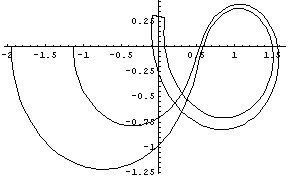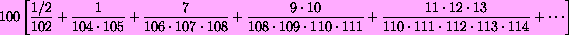# Noam's Mathematical Miscellany:

## Geometrical morsels

A5: New observations on an old puzzle

cos1, cos2: Dissections showing the Law of Cosines c2 = a2 + b2 - 2ab cos C for a triangle with an obtuse (PS, PDF) or acute (PS, PDF) angle at C. In the former case, attach two copies of the triangle to a square on side c, then translate and remove them so the remaining area can be dissected into squares on sides a,b and two parallelograms each of area  -ab cos C. In the latter case, attach two parallelograms of area  ab cos C  and three copies of the triangle to a square on side c, then translate and remove the triangles to leave behind disjoint squares on sides a,b. Either of these generalizes an ancient dissection picture for the Pythagorean theorem, which can be recovered by making C a right angle.

Added Feb.2004: not surprisingly, it turns out that I was not the first to discover these dissections when I found them about 10 years ago -- at least in the obtuse case, where one doesn't need to borrow a third triangle. Douglas Rogers writes:

Here is an appearance from Math. Gaz., 12 (1924-1925), 107, I happen to have on file. As a tiling pattern, this picture has a long history: Leonhard Sohncke has it in connection with his crystallographic studies in Crelle's Journal in 1873 (mentioned, for example, in the book of Granbaum and Shephard on tilings, in 1987); but as a floor pattern it may well be very early. [...] For more on this dissection for the Law of Cosines, do please see Greg Frederickson's book Dissections: Plain and Fancy, pp. 38-39, where he cites work of Rudolf Hunger and Erwin Dintzl (the book was reissued in paperback in 2003 by the Cambridge University Press).

rect1, rect2: Beware of dissection proofs! This picture purports to show the same two pairs of triangles fit into the same 9-by-20 rectangle in four ways, in each case with two rectangles left over. In each case, then, the sum of the two rectangles' areas should be the difference between 9*20 and the sum of the areas of the four triangles. But in each of the four dissections, the rectangles have a different total sum: 88, 89, 90, 91. Here the two rectangles of area 44 are decomposed into five polyominoes that can be recombined to form the remaining rectangle pairs with one, two, or three unit-square holes. Do we have a proof that 88=89=90=91?
The 88=89=90 part is a known doubling of an illusion that goes back to Dudeney. In February 2004, Joe Buhler showed me a physical realization by Helaman Ferguson: an 8-by-21 rectangle that can be filled by four triangles and a number of polyominoes, leaving room for zero, one, or two unit squares. While trying to fit the second square in, I tried a configuration that corresponds to the fourth picture, and failed miserably; but the next day I found that this variation with a 9-by-20 works.

## Puzzles

1. Natural numbers a,b,c,d between 1 and a xillion are chosen at random, with each of the xillion-to-the-fourth-power possible quadruples equally likely. What is the probability that gcd(a,b)=gcd(c,d) ?
SOLUTION

2. Circles C and C' meet at points P and Q. A line tangent to both circles meets them at points A and A'. Consider the triangle T whose vertices are P, Q, and the midpoint of the line segment AA'. Given the radii r and r' of C and C', how large can the area of T be?
SOLUTION

3. Using each of digits 1, 2, 3 once, and only basic arithmetic operations, write a formula for 19. (The only "basic arithmetic operations" here are add/subtract/divide/multiply, powers and roots, and factorials; in particular, no decimals or greatest-integer functions.)
SOLUTION

4. If that was too easy (or too silly), use 2, 5, 7 and basic arithmetic to write 181.
SOLUTION

5. If 32 Knights are placed on all the light squares of a standard 8-by-8 chess board, no two Knights observe each other. Likewise, of course, if we use all the dark squares instead. It has long been known that 32 is the maximum, and that those are the only configurations that attain it [Newman, Patenaude, and Greenberg: Problem E1585 and solutions, Amer. Math. Monthly 71, Feb.1964].
A) Instead of requiring each Knight to be unobserved, suppose we relax the condition by allowing each Knight to be either unobserved or observed by just one other Knight. How many Knights can the 8-by-8 board accommodate now?
B) What if we require that each Knight be observed by exactly one other Knight?
SOLUTION

6. [From a recent Mathematics GRE] Is there a well-ordered uncountable set of real numbers?
(Recall that a set is well-ordered when every nonempty subset has a least element. That is, S is well-ordered when every nonempty subset S' of S has an element x smaller than every other element of S'. Every finite set is well-ordered. The classic example of an infinite well-ordered set is {1,2,3,...}, which is infinite but of course only countable.)
SOLUTION

7. [A problem I contributed to the 1988 Qualifying Exam at Harvard]
Prove that a finite group of 8k elements (k=1,2,3,...) must have at least five conjugacy classes.
SOLUTION

8. For positive x<1, consider the alternating sum
S(x) = x - x2 + x4 - x8 + x16 - x32 + - ...
Does S(x) approach a limit as x approaches 1 from below, and if so what is this limit?
SOLUTION
9. The following picture appeared without explanation on a web page for John Derbyshire's Prime Obsession. What does it represent?SOLUTION
10. A unit cube has twelve pairs of vertices at distance 1 (the sides) and four pairs at distance 31/2 (the body diagonals). Must every of eight points with the same configuration of twelve unit distances and 4 distances of 31/2 be a unit cube? (NB it is not required that the remaining twelve distancess equal 21/2.)
SOLUTION
11. Round to the nearest integer:SOLUTION

12. What does each of the following pairs share (besides having the same sum)?
 (3, 3, 12) = (1, 8, 9) (4, 8, 9, 21) = (3, 7, 14, 18) (4, 7, 21, 36) = (1, 12, 27, 28) (5, 7, 10, 14, 27) = (3, 6, 15, 18, 21) (5, 85, 85, 169, 425) = (13, 17, 125, 289, 325) (17, 21, 24, 48, 54, 238) = (3, 4, 14, 119, 126, 136)
SOLUTION

13. (In honor of Elwyn Berlekamp and the Mathematical Sciences Research Institute) Solve the cryptarithm
MS / RI = .ELWYNELWYNELWYNELWYN...
[That is, find distinct digits M,S,R,I,E,L,W,Y,N that make MS/RI=.ELWYNELWYNELWYNELWYN... a true equation.]
SOLUTION

## PostScript tricks

eratosthenes: Runs the Sieve of Eratosthenes to find and print all primes up to 2000. (Here's the short PostScript file to prove I'm not cheating.)

expsum: A surprisingly short PostScript file to generate this picture; go here for the explanation.

ford: A PostScript file that recursively draws Ford circles: a system of non-overlapping circles each tangent to infinitely many others and to a given line. (The line and Ford circles are in black; see below for the blue semicircles.) You can inspect the Postscript file to see that I'm actually recursing rather than getting the circles from a formula (see below) or list. Suitable arcs on these circles are used as contours of integration in obtaining Rademacher's series for the partition function and related formulas. If the line segment is identified with the interval [0,1], the circles are tangent to the segment at the Farey fractions m/n and have diameters 1/n2. The blue semicircles are orthogonal to the Ford circles at their points of tangency. If we identify the right half-plane with the Poincaré model of the hyperbolic plane, the blue semicircles become hyperbolic lines, and the Ford circles are horocycles.

gasket1, gasket2: A similar but more complicated recursion. These PostScript files recursively draw Apollonian gaskets (gasket1 with trilateral symmetry, gasket2 showing a closeup of a three-cornered wedge). Again you can consult the PostScript files here and here to see that I'm actually recursing rather than specifying each circle's position individually. To draw more and smaller circles, increase the threshold M (currently 150).

1dca: Henry Cohn's PostScript code for pictures of 1-dimensional cellular automata. For instance, this file shows the mysterious “Rule 30” in action.

## One-page papers

A8: The isomorphism between the groups GL4(Z/2Z) and A8 via the projective plane of order 2.
LaTeX, DVI, PDF

abel: Elementary calculus proof of Abel's formula for the power series of the inverse function of ye-y and of arbitrary powers of this function (which can also be obtained as a scaled limit from the next paper).
LaTeX, DVI, PDF

catalan: Elementary calculus proof of the formula for the coefficients of the power series of the inverse function of y(1-y)t (generalized Catalan numbers) and of arbitrary powers of this function.
LaTeX, DVI, PDF

catalan2: Yet another proof, one that uses only basic power-series manipulations (no derivatives, even), but at the expense of requiring that the answer be known or guessed in advance.
LaTeX, DVI, PDF
Again a proof for Abel's formula can be obtained as a scaled limit; the resulting argument was given by Richard Stanley in his Enumerative Combinatorics (Volume 2, bottom of p.28), and Stanley's method is the key ingredient in this proof for the generalized Catalan numbers.

E8: At an AIM workshop, Griess asked for all known proofs of the result, apparently first proved by Mordell in 1938, that the E8 root lattice is the unique even unimodular lattice of rank 8 (the smallest rank possible). I realized the next day that the n=8 case of my characterization of the Zn lattice could be used to construct yet another proof. This proof is elementary except for the use of theta functions in my Zn paper; even so, the argument is arguably still simpler than in previous theta-function proofs.
LaTeX, DVI, PDF

hilbert: The parametrization of Pythagorean triples (integer solutions of x2 + y2 = z2) as a special case of Hilbert's Theorem 90.
[The same method applies to x2 + axy + by2 = z2 for any constants a, b except those for which a2 - 4b is a square; for instance, Hilbert 90 can be used to parametrize rational triangles with a 60- or 120-degree angle.]
LaTeX, DVI, PDF
Thanks to F.Lemmermeyer for recognizing the Pythagorean case of this proof from the beginning of Olga Taussky's “Sums of Squares”, American Mathematical Monthly, Vol.77 (1970), 805-830. Lemmermeyer notes that Ono gives the same proof in his book Variations on a Theme of Euler: Quadratic Forms, Elliptic Curves and Hopf Maps. There's no reference to O.Taussky there either, so presumably Ono also discovered this independently.

hsgraph: Outline of a different proof of existence and uniqueness of the Hoffman-Singleton graph (the regular graph on 50 vertices of degree 7, diameter 2, and girth 5).
LaTeX, DVI, PDF

innaz: A different derivation of Euler's integral formula for n!, by Inna Zakharevich (Harvard '06).
LaTeX, DVI, PDF
corrected 10/2003 to fix a typo spotted by David Leep; fortunately the derivation is unaffected.

mahler: A direct proof, using generating functions, of Mahler's theorem on continuous p-adic maps.
LaTeX, DVI, PDF

pi10: Why is Pi so close to the square root of 10?
[Published as a “boxed filler” in the American Mathematical Monthly, Vol.110 #7 (Aug.-Sep. 2003), page 592]
LaTeX, DVI, PDF

quickie: I proposed this problem to the American Math Monthly. The Problems editor rejected it on the advice of both referees -- one of whom thought the problem unfairly difficult, the other thought it trivial! What do you think?
LaTeX, DVI, PDF